# Study Guide

## Field 018: Chemistry Sample Multiple-Choice Questions

The following reference material will be available to you during the test:

Periodic Table#### REFLECTING ON AND CONSTRUCTING SCIENTIFIC KNOWLEDGE

##### Objective 001Understand the principles and procedures of scientific inquiry.

1. Use the information below to answer the question that follows.

A researcher carried out a reaction between 0.010 M reactant X and excess reactant Y. The researcher measured the concentration of product Z at 60-second intervals. The researcher's results are shown in the graph below.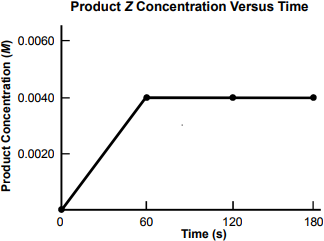The graph is a line graph with one data plot. Its title is "Product Z Concentration Versus Time." The vertical axis is labeled Product Concentration, in M, with values marked from 0 to 0.0060 in increments of 0.0020. The horizontal axis is labeled Time, in seconds, with values marked from 0 to 180 in increments of 60. The line graph has data points at (0, 0), (60, 0.0040), (120, 0.0040), and (180, 0.0040).

The researcher concludes from these data that the reaction rate was 6 point 7 times 10 to the negative fifth power M per second. Which of the following is the best evaluation of the validity of the researcher's conclusion?

1. It is invalid because the chosen time interval of 60 seconds was not short enough to allow for an accurate measurement of the rate of this reaction.
2. It is valid because the leveling off of the product concentration curve indicates the reaction was complete at 60 seconds.
3. It is invalid because the rate should have been calculated by dividing the final product concentration by the full 180-second duration of the experiment.
4. It is valid because reaction rate is an intensive property of the reactants and products involved in the reaction.
Correct Response: A.

##### Objective 002Apply knowledge of methods and equipment used in scientific investigations.

2. A student has to measure out 9.40 mL of a liquid and selects a 100 mL graduated cylinder. To improve the accuracy of the measurement, it would be most effec­tive to:

1. take the average of multiple measurements using the graduated cylinder.
3. estimate the measurement obtained from the graduated cylinder to an additional significant figure.
Correct Response: D.

##### Objective 003Understand the nature of scientific thought, inquiry, and history.

3. Which of the following is an illustration of the use of verifiable evidence in the practice of science?

1. Thinkers in ancient India proposed that atoms came in two forms, soft or rough, which caused them to combine in pairs, forming the basis for all matter.
2. Ernest Rutherford hypothesized that atoms must have dense cores, since some alpha particles, when shot at gold foil, passed through and others were greatly deflected.
3. Niels Bohr reasoned that an atom's electrons orbiting the nucleus would have to radiate energy in a manner consistent with the law of conservation of energy.
4. Democritus proposed the idea of the atom when the smell of baking bread caused him to question how the smell traveled to one's nose from a distance.
Correct Response: B.

##### Objective 004Understand the relationship of chemistry to contemporary, historical, technological, and societal issues.

4. Scientific claims published in professional journals are generally considered more credible than those posed through the mass media. This is true primarily because professional journals:

1. ensure that every investigation is repeated and the results indepen­dently verified before a paper is published.
2. direct their content to a more narrow readership with highly specialized interests.
3. maintain their editorial objectivity by refusing to accept paid advertising.
4. subject researchers' methodologies and conclusions to peer review prior to publication.
Correct Response: D.

##### Objective 005Understand interrelationships among the physical, life, and earth/space sciences and their connections to mathematics and technology.

5. The most important similarity between the mole in chemistry and the light year in astronomy is that these units:

1. provide accurate physical models of natural phenomena.
2. have been refined since first being introduced as more data were collected.
3. represent combined laws worked out by numerous researchers.
4. convert extremely large- or small-scale phenomena into values that are easier to work with.
Correct Response: D.

#### USING INORGANIC CHEMISTRY

##### Objective 006Apply the rules of chemical nomenclature and notation.

6. Which of the following is the correct notation for the calcium-44 isotope existing as a 2+ ion?

1.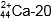2.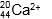3.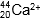4.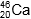Correct Response: C.

##### Objective 007Understand atomic and molecular structure and bonding.

7. Which of the following substances is likely to exhibit the highest boiling point due to intermolecular forces?

1. CH3OH
2. CH3SH
3. CH3F
4. CH2O
Correct Response: A.

##### Objective 008Apply the mole concept and the principles and methods of stoichiometry.

8. Which of the following quantities contains the greatest number of moles?

1. 30 g of N2
2. 35 g of NH3
3. 60 g of NaCl
4. 75 g of CaO
Correct Response: B.

##### Objective 009Apply knowledge of chemical equilibrium and reaction rates.

9. Use the information below to answer the question that follows.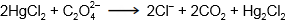A chemical equation is shown and reads as follows; Two capital H lowercase g Capital C lowercase l subscript two reacts with capital C subscript two capital O sup two minus subscript four to produce two capital C lowercase l sup minus and two capital C capital O subscript two and capital H lowercase g subscript two capital C lowercase l subscript two.

The reaction above was carried out with various starting concentrations of the two reactants, and the initial reaction rate was determined. The experimental results are recorded in the table below.

Trial [HgCl2](M) open bracket capital C subscript two capital O sup two minus subscript 4 close bracket(M) Initial Rate (M/s)
1 0.05 0.15 8.75 × 10−6
2 0.05 0.30 3.50 × 10−5
3 0.10 0.15 1.75 × 10−5
4 0.10 0.30 7.00 × 10−5

Which of the following rate laws is consistent with the experimental data?

1.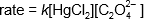2.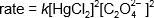3.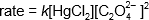4.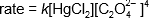Response A. rate equals k open bracket capital H lowercase g capital C lowercase l subscript two close bracket open bracket capital C subscript two capital O sup two minus subscript 4 close bracket

Response B. rate equals k open bracket capital H lowercase g capital C lowercase l subscript two close bracket sup two open bracket capital C subscript two capital O sup two minus subscript 4 close bracket sup 2

Response C. rate equals k open bracket capital H lowercase g capital C lowercase l subscript two close bracket open bracket capital C subscript two capital O sup two minus subscript 4 close bracket sup 2

Response D. rate equals k open bracket capital H lowercase g capital C lowercase l subscript two close bracket open bracket capital C subscript two capital O sup two minus subscript 4 close bracket sup 4

Correct Response: C.

##### Objective 010Understand the principles and applications of acid-base chemistry.

10. Which of the following is a characteristic of methanoic acid (HCOOH) that makes it a suitable component of a buffer?

1. It changes color when it undergoes ionization.
2. Its ionization is incomplete.
3. It has two ionizable hydrogens.
4. It can ionize to produce both hydrogen and hydroxide ions.
Correct Response: B.

##### Objective 011Understand the principles and applications of electrochemistry.

11. Use the reaction below to answer the question that follows.

A chemical equation reads as follows;Capital A solid reacts with capital B superscript positive one, aqueous, to produce capital A superscript positive one, aqueous, and capital B solid.

The overall reaction of an electro­chemical cell is shown above. The cell potential is 1.0 V when the cell is at 25°C and the solution concentrations are 1.0 M. Which of the following changes would result in an increase in cell potential?

1. increasing the concentration of B+(aq)
2. increasing the concentration of A+(aq)
3. increasing the surface area of the B(s) electrode
4. increasing the surface area of the A(s) electrode
Correct Response: A.

##### Objective 012Understand qualitative analysis.

12. A soluble ionic compound is thought to contain either sodium or calcium as the cation. Which of the following solutions could be added to an aqueous sample of the compound to determine the identity of the cation?

1. hydrochloric acid
2. nitric acid
3. potassium bromide
4. potassium carbonate
Correct Response: D.

#### USING PHYSICAL CHEMISTRY

##### Objective 013Understand chemical thermodynamics and thermochemistry.

13. Use the information below to answer the question that follows.

A chemical equation is shown and reads as follows; Capital H capital B lowercase r, aqueous, reacts with capital N lowercase a capital O capital H, aqueous, to produce capital N lowercase a capital B lowercase r, aqueous, and capital H subscript 2 capital O, liquid.

The reaction shown above is studied in a constant-pressure calorimeter with a heat capacity of 500 J/°C. In the experiment, 100 mL of 0.10 M HBr and 100 mL of 0.10 M NaOH are mixed. The entire system, including both solutions, started at 20.00°C, and the final temperature was 20.42°C. Assuming additive volumes, no heat loss to the surroundings, solution densities of 1.00 g/mL, and solution specific heats of 4.184 J/g•°C, what is the approximate value of ΔH for the reaction?

1. −35 kJ/mol
2. −40 kJ/mol
3. −56 kJ/mol
4. −123 kJ/mol
Correct Response: C.

##### Objective 014Apply methods for measuring the physical properties of solids, liquids, and gases.

14. An unknown substance is a solid at room temperature. The substance is soluble in water, but the aqueous solution does not conduct electricity. Attempts to measure the melting point of the substance are unsuccessful, as a chemical reaction occurs prior to melting. Which of the following substances is most consistent with this description of physical properties?

1. CH3OH
2. (NH4)2SO4
3. MgCO3
4. C6H12O6
Correct Response: D.

##### Objective 015Apply knowledge of the kinetic molecular theory to the states of matter, phase changes, and the gas laws.

15. Use the graph below to answer the question that follows.The graph is a line graph with one data plot. Its title is "Heating Curve for a Substance." The vertical axis is labeled "Temperature," and the horizontal axis is labeled "Heat Added." The data line begins somewhat above the origin and ascends stepwise in a series of segments that alternate between a 45° angle and horizontal. The segments are labeled A through D, beginning with an angled segment labeled A.

The graph above is the heating curve for a substance covering its solid, liquid, and gaseous phases. Which segment of the graph gives information about this substance's molar heat of fusion?

1. segment A
2. segment B
3. segment C
4. segment D
Correct Response: B.

##### Objective 017Understand quantum mechanics.

16. According to quantum theory, an atomic orbital represents:

1. a region of space around the nucleus where the probability of finding an electron is high.
2. the three-dimensional path that a given electron follows around the nucleus.
3. the particular distance from the nucleus that a given electron must maintain.
4. a particular point around the nucleus where a given electron must be located.
Correct Response: A.

##### Objective 018Understand the basic principles and methods of spectroscopy.

17. A food scientist has a sample of a plant oil and wants to determine if the oil contains saturated or unsaturated fatty acids. Which of the following spectroscopic techniques would be most useful for this purpose?

1. ultraviolet spectroscopy
2. visible spectroscopy
3. infrared spectroscopy
4. mass spectroscopy
Correct Response: C.

#### USING ORGANIC CHEMISTRY AND BIOCHEMISTRY

##### Objective 019Understand the structure and nomenclature of organic compounds.

18. Which of the following molecules can have both cis and trans geometrical isomers?

1. CH3(CH2)2CH3
2. CH2CHCH2CH3
3. CH3CCCH3
4. CH3CHCHCH3
Correct Response: D.

##### Objective 020Understand organic reactions of major functional groups.

19. Use the chemical equation below to answer the question that follows.

Which of the following kinds of chemical reaction is represented by the equation above?

2. condensation
3. elimination
4. substitution
Correct Response: A.

##### Objective 022Understand biochemical reactions and processes.

20. In living cells, nonspontaneous metabolic reactions are made thermodynamically possible primarily through:

1. direct interaction of the bonds in carbohydrates with those in the molecules involved in the metabolic reaction.